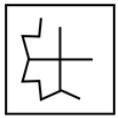• Save

# Non Verbal Reasoning Questions and Answers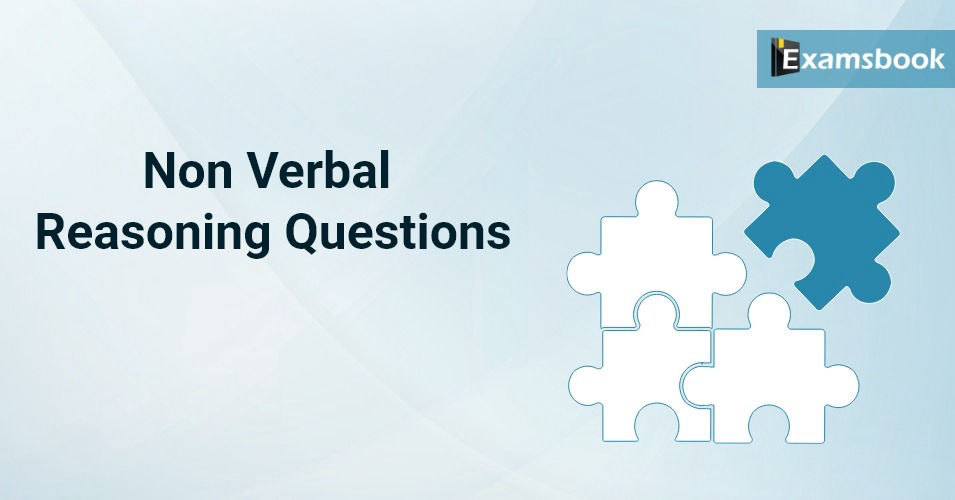In the present competitive examinations, the importance of each mark has increased so much that one mark makes us stand ahead of thousands of students. Keeping this in mind, Examsbook has prepared a blog of non-verbal reasoning with MCQ questions for competitive exams, in which all point counting figures, identifying figures, completing figures, paper folding, water mirror, mirror Questions on Image, Cube, Cuboid and other non-verbal reasoning are included.

By engaging with this Non-Verbal Reasoning blog, you can find the right answers which are asked in non-verbal section in all competitive exams like SSC, bank, Railway, Delhi Police, BSF Army, CISF, NDA, Navy, CET Exams.

To get assured success in your competitive exams, practice online  here with free practice tests also as well as these questions which will prove to be best for you. Click here to Download mobile app.

## Non Verbal Reasoning Questions

Q :

How many triangles are there in the given figure?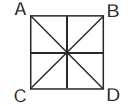(A) 16

(B) 8

(C) 14

(D) 11

Select the figure from among the given options that can replace the question mark (?) in the following series.

Q :

Question figure.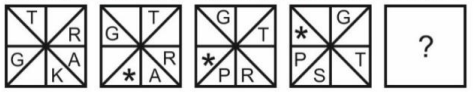(A)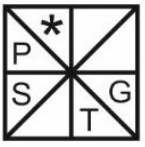(B)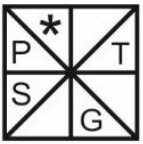(C)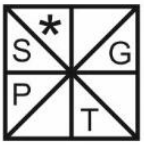(D)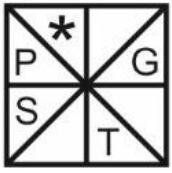Directions: In each of the following questions which of the answer figures is exactly the mirror image of the given figure when the mirror is held at AB?

Q :

Question Figure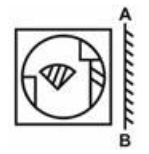(A)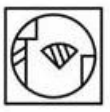(B)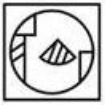(C)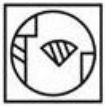(D)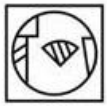Direction : Which of the answer figures is exactly the water image of the given figure?

Q :

Question Figure: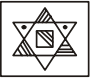(A)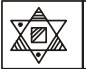(B)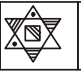(C)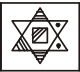(D)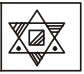Directions : In which answer figure is the question figure embedded?

Q :

Question figure.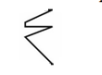(A)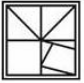(B)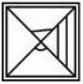(C)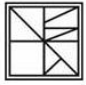(D)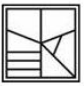Select the figure from the options that can replace the question mark (?) and complete the pattern. (Rotation is not allowed)

Q :

Question figure.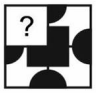(A)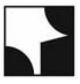(B)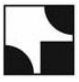(C)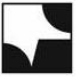(D)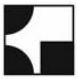The sequence of folding a piece of paper and the manner in which the folded paper has been cut is shown in the following figure. How would this paper look when when unfolded?

Q :

Question Figure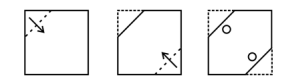(A)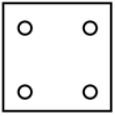(B)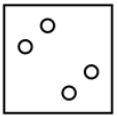(C)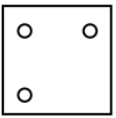(D)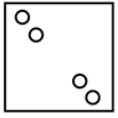Q :

A cube is coloured blue on all faces. It is cut into 64 small cubes of equal size. How many cubes have only one face coloured?

(A) 4

(B) 8

(C) 16

(D) 24

Q :

How many triangles are there in the given figure?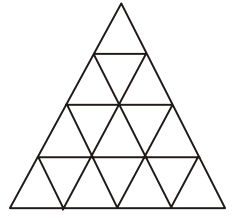(A) 29

(B) 27

(C) 23

(D) 30

Select the figure from among the given options that can replace the question mark (?) in the following series.

Q :

Question figure.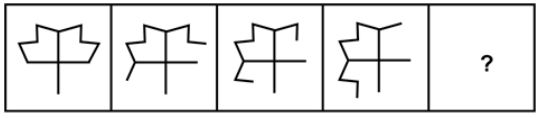(A)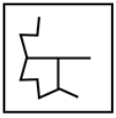(B)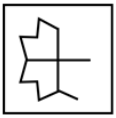(C)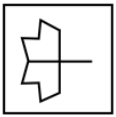(D)